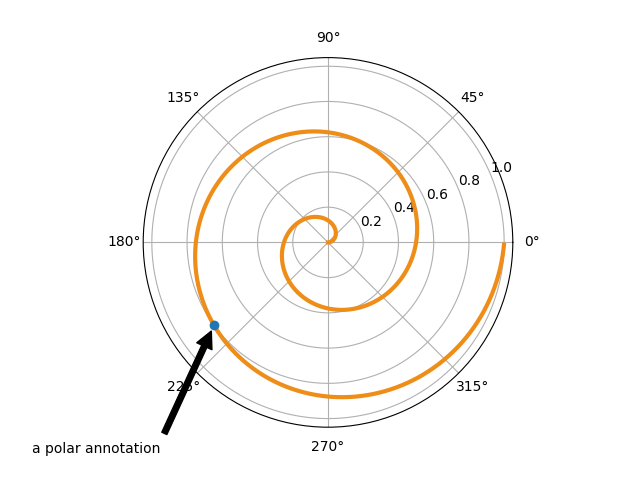# Annotation Polar¶

This example shows how to create an annotation on a polar graph.

For a complete overview of the annotation capabilities, also see the annotation tutorial.

import numpy as np
import matplotlib.pyplot as plt

fig = plt.figure()
r = np.arange(0,1,0.001)
theta = 2 * 2*np.pi * r
line, = ax.plot(theta, r, color='#ee8d18', lw=3)

ind = 800
thisr, thistheta = r[ind], theta[ind]
ax.plot([thistheta], [thisr], 'o')
ax.annotate('a polar annotation',